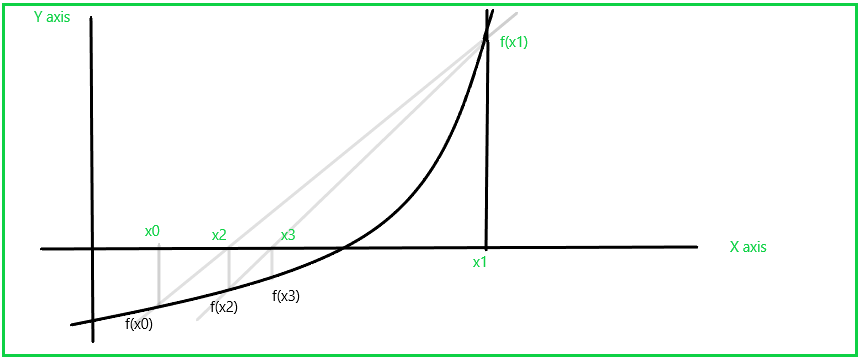# Secant Method of Numerical analysis

• Difficulty Level : Medium
• Last Updated : 18 Oct, 2021

Secant method is also a recursive method for finding the root for the polynomials by successive approximation. It’s similar to the Regular-falsi method but here we don’t need to check f(x1)f(x2)<0 again and again after every approximation. In this method, the neighbourhoods roots are approximated by secant line or chord to the function f(x). It’s also advantageous of this method that we don’t need to differentiate the given function f(x), as we do in Newton-raphson method.Figure – Secant Method

Now, we’ll derive the formula for secant method. The equation of Secant line passing through two points is :Here, m=slope

So, apply for (x1, f(x1)) and (x0, f(x0))

` Y - f(x1) = [f(x0)-f(x1)/(x0-x1)] (x-x1)   Equation (1)`

As we’re finding root of function f(x) so, Y=f(x)=0 in Equation (1) and the point where the secant line cut the x-axis is,

`x= x1 - [(x0 - x1)/ (f(x0) - f(x1)]f(x1) .`

We use the above result for successive approximation for the root of function f(x). Let’s say the first approximation is x=x2:

`x2= x1 - [(x0 - x1)/ (f(x0)-f(x1))]f(x1)`

Similarly, the second approximation would be x =x3:

`x3= x2 - [(x1-x2)/ (f(x1)-f(x2))]f(x2)`

And so on, till kth iteration,

`xk+1= xk - [(xk-1 - xk) / (f(xk-1) - f(xk))]f(xk)`

Note: To start the solution of the function f(x) two initial guesses are required such that f(x0)<0 and f(x1)>0. Usually it hasn’t been asked to find, that root of the polynomial f(x) at which f(x) =0. Mostly You would only be asked by the problem to find the root of the f(x) till two decimal places or three decimal places or four etc.

• The speed of convergence of secant method is faster than that of Bisection and Regula falsi method.
• It uses the two most recent approximations of root to find new approximations, instead of using only those approximations which bound the interval to enclose root

• The Convergence in secant method is not always assured.
• If at any stage of iteration this method fails.
• Since convergence is not guaranteed, therefore we should put limit on maximum number of iterations while implementing this method on computer.

Example-1 :
Compute the root of the equation x2e–x/2 = 1 in the interval [0, 2] using the secant method. The root should be correct to three decimal places.

Solution –
x0 = 1.42, x1 = 1.43, f(x0) = – 0.0086, f(x1) = 0.00034.

Apply, secant method, The first approximation is,
x2 = x1 – [( x0 – x1) / (f(x0) – f(x1)]f(x1
= 1.43 – [( 1.42 – 1.43) / (0.00034 – (– 0.0086))](0.00034)
= 1.4296
f(x2) = – 0.000011 (–ve)

The second approximation is,
x3 = x2 – [( x1 – x2) / (f(x1) – f(x2))]f(x2
= 1.4296 – [( 1.42 – 1.4296) / (0.00034 – (– 0.000011](– 0.000011)
= 1.4292
Since, x2 and x3 matching up to three decimal places, the required root is 1.429

Example-2 :
A real root of the equation f(x) = x3 – 5x + 1 = 0 lies in the interval (0, 1). Perform four iterations of the secant method.

Solution –
We have, x0 = 0, x1 = 1, f(x0) = 1, f(x1) = – 3
x2 = x1 – [( x0 – x1) / (f(x0) – f(x1))]f(x1
= 1 – [ (0 – 1) / ((1-(-3))](-3)
= 0.25.

f(x2) = – 0.234375

The second approximation is,
x3 = x2 – [( x1 – x2) / (f(x1) – f(x2))]f(x2
=(– 0.234375) – [(1 – 0.25)/(–3 – (– 0.234375))](– 0.234375)
= 0.186441

f(x3)

The third approximation is,
x4 = x3 – [( x2 – x3) / (f(x2) – f(x3))]f(x3)
= 0.186441 – [( 0.25 – 0.186441) / ( – 0.234375) – (0.074276) ](– 0.234375)
= 0.201736.

f(x4) = – 0.000470
The fourth approximation is,
x5 = x4 – [( x3 – x4) / (f(x3) – f(x4))]f(x4)
= 0.201736 – [( 0.186441 – 0.201736) / (0.074276 – (– 0.000470)](– 0.000470)
= 0.201640

My Personal Notes arrow_drop_up
Recommended Articles
Page :